# Independent Complex Samples t-Test - Comparing Two Means

Where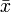$\bar{x}$ and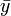$\bar{y}$ are the two means and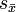$s_\bar{x}$ and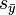$s_\bar{y}$ are their Standard Errors, the test statistic is:$t=\frac{\bar{x}-\bar{y}}{\sqrt{s^2_\bar{x} + s^2_\bar{y}}}$,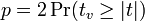$p = 2\Pr(t_v \ge |t|)$,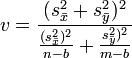$v = \frac{(s^2_\bar{x} +s^2_\bar{y})^2}{\frac{(s^2_\bar{x})^2}{n-b}+\frac{s^2_\bar{y})^2}{m-b} }$
the groups have sample sizes of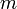$m$ and$n$ respectively (or effective sample sizes if a design effect has been specified),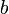$b$ is 1 if Bessel's correction is selected for Means in Statistical Assumptions and 0 otherwise.